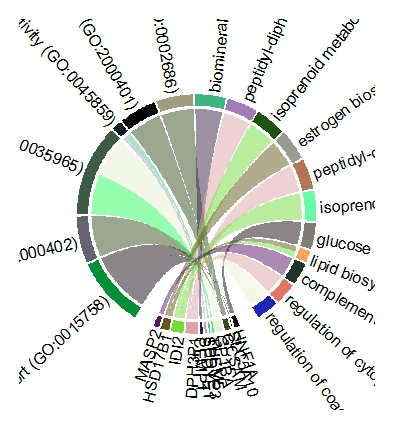circlize output image problem
0
0
Entering edit mode
4 months ago

Hi I plotted chord diagram by the circlize R package In accordance with its tutorial (https://jokergoo.github.io/circlize_book/book/advanced-usage-of-chorddiagram.html). But the plots are drawn incompletely.chordDiagram(day0)
chordDiagram(day0, annotationTrack = c("name", "grid"),
annotationTrackHeight = c(0.03, 0.01))
circos.track(track.index = 1, panel.fun = function(x, y) {
circos.text(CELL_META$xcenter, CELL_META$ylim, CELL_META\$sector.index,
facing = "clockwise", niceFacing = TRUE, adj = c(0, 0.5)) }, bg.border = NA)


The labels are not in the photo correctly and completely. I appreciate if anybody share his/her comment with me.

circlize chord diagram • 161 views